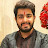Discriminants | Algebra | Chegg TutorsDiscriminants | Algebra | Chegg Tutors

The discriminant of an equation gives an idea of the number of roots and the nature of roots of the equation. In other words, it “discriminates” between the possible solutions. The discriminant is the expression found under the square root part of the quadratic formula x = -b ± √b2 – 4ac/2a (that is, D = b2 – 4ac. The value of (b2 – 4ac) tells how many solutions, roots, or x-intercepts the quadratic equation will have.
If (b2 – 4ac) is greater than 0, there are two real solutions.
If (b2 – 4ac) is equal 0, there is one real solution.
If (b2 – 4ac) is lesser than 0, there are no real solutions, but there are two complex imaginary solutions.
To find the solutions, manipulate the quadratic equation to standard form (ax2 + bx + c = 0), determine a, b, and c, and plug those values into the discriminant formula.

———-

Algebra tutoring on Chegg Tutors

Learn about Algebra terms like Discriminants on Chegg Tutors. Work with live, online Algebra tutors like Chris W. who can help you at any moment, whether at 2pm or 2am.

Liked the video tutorial? Schedule lessons on-demand or schedule weekly tutoring in advance with tutors like Chris W.

———-

About Chris W., Algebra tutor on Chegg Tutors:

University of Pennsylvania, Class of 2007
Math, Computer Science major

Subjects tutored: LaTeX, ACT (math), SAT (math), SSAT (math), Information Technology, Discrete Math, SAT II Mathematics Level 2, Basic Math, Web Design, Numerical Analysis, Geometry, Pre-Calculus, SAT II Mathematics Level 1, GRE, Pre-Algebra, Software Engineering, Set Theory, Applied Mathematics, Computer Certification and Training, Number Theory, Statistics, Linear Algebra, PSAT (math), Linear Programming, Computer Science, Calculus, Trigonometry, Algebra, and Geometry (College Advanced)

TEACHING EXPERIENCE
Over 7 years of experience teaching math at 3 universities and a community college. Courses ranged from Intermediate Algebra to Calculus II and class sizes varied from 2 to over 200 students. Tutoring since 2000 formally and informally, individually and in groups, for courses from Geometry to Differential Equations. Please note that I generally will not be available for audio and video in live lessons but my experience has been that audio and video aren’t really needed.

EXTRACURRICULAR INTERESTS
Hiking, reading, video games, playing the guitar and piano.

Want to book a private lesson with Chris W.?

———-

Like what you see? Subscribe to Chegg’s Youtube Channel:

http://bit.ly/1PwMn3k

———-

Visit Chegg.com for purchasing or renting textbooks, getting homework help, finding an online tutor, applying for scholarships and internships, discovering colleges, and more!

https://chegg.com

———-

Want more from Chegg? Follow Chegg on social media:

http://instagram.com/chegg

algebra tutors,Chegg,Chegg Study,Online Tutoring,Homework Help,Discriminants,Algebra,Chegg Tutors

algebratutors.org

1.Taurean Kartik says: